## Introduction

One of many astonishing properties of graphene is its weak electron-phonon (e-p) coupling. In normal conductor, e-p scattering quickly dominates as the temperature increases, resulting in a diminishing carrier mobility. In contrast, e-p scattering in graphene is negligible even at room temperature1,2. Consequently, graphene has so far the highest mobility at room temperature among all the other materials3,4,5. Because of its high mobility, applications in high speed transistors6,7,8 and in highly conductive interconnects have been foreseen. Recently, significant interest has grown in using graphene as a photodetector by exploiting its weak e-p interactions9,10,11,12,13,14,15,16. One type of such detectors is known as hot electron bolometer (HEB). Potentially, graphene HEB is very sensitive and extremely fast at the same time17, due to its small electron heat capacity and the low thermal conductance between the electron and phonon gases. Several attempts have been made in realizing graphene HEBs and the main goal is to obtain a large temperature coefficient for the resistance of graphene. For example, an energy gap was introduced either by applying a strong magnetic field to form Landau levels11, by a dual-gated bilayer graphene13 or alternatively by using a superconducting tunnel junction14. In a different approach, the electronic temperature was measured by means of the noise thermometry instead of the resistance18,19,20. However, a simple resistive readout would be preferred in practice. Furthermore, it would be highly desired if a graphene HEB can be made without acquiring high quality graphene films and dedicated micro-fabrication process and can operate without a magnetic field.

This present work demonstrates a new approach for implementing a graphene HEB. The main key is to drive the electronic system into the strong localization regime by adding disorder. Note that disorder here in general denotes the scattering potentials of various forms, such as lattice defects, charge impurities, etc. The divergence of the resistance at low temperature will allow for sensitive temperature measurement. The high resistance, together with an as-grown Boron Nitride (BN) tunneling barrier between graphene and electrical contacts, also reduces thermal dissipation out of the electron gas via diffusion. The bolometer fabricated with such method is found highly sensitive and with a very low background noise. Moreover, this approach does not require graphene with high mobility. The device structure with merely a graphene bar in contact with two electrodes can be easily fabricated with standard photo-lithography technique and extended into a 2D detector array.

## Results

The samples used in this study were Graphene/BN bilayer grown by CVD method21. In this method, monolayer BN is first grown on copper foil, followed by growth of graphene on top. Depending on the growth condition, the quality of graphene films can be easily controlled and finely tuned. In order to obtain the defective graphene films, a high carbon precursor flux and a low growth temperature were employed in promoting dense nucleation22. Films grown by this means exhibit significant defects, as evidenced by a strong D peak in the Raman spectrum in Fig. 1(a)23,24.

Our bolometer devices consist of a 5 μm wide BN/Graphene ribbon on the metal electrodes, see Fig. 1(b) and (c). The graphene film is separated from the electrodes by the BN layer, which would be acting as a tunneling barrier. Its role is to increase the electrical contact resistance, hence the thermal resistance. The result is better thermal isolation. An estimation and discussion of the thermal resistance will be given later. The resistance of such graphene films increases with the decreasing temperature and then diverges at low temperature, shown in Fig. 1(d). We plot the resistance R against T−1/3 in a semilog scale, where a linear dependence spans from the lowest temperature of 1.5 K to 300 K. The data suggest that electrons are strongly localized and the transport is dominated by the so-called variable range hopping (VRH)25. For VRH, the resistivity ρ(T) exp[(T0/T)1/3]. Here, T0 is a characteristic temperature, T0 = 12/πkBg(EF2, where kB is the Boltzmann constant, g(EF) the density of states at the Fermi level EF, ξ the localization length. We have performed a linear fit for the ln R versus T−1/3 plot. The slope of the fit yields a localization length ξ of 50 nm. Both the Raman results and the transport data indicate that the graphene film is disordered. The sharp increase of the resistance at low temperature due to localization is the key ingredient of our bolometer, as the responsivity of our bolometer is proportional to the slope of the RT curve, dR/dT. Fig. 1(e) shows dR/dT as a function of temperature. At 2 K, dR/dT reaches 22 kΩ/K. Moreover, a high electrical resistance means a high thermal resistance, which prevents heat from dissipation via diffusion and also helps the responsivity.

To see how the bolometer responses to heating, we apply a d.c. bias current Idc to generate heat in the electron gas. As shown in Fig. 2(b), the differential resistance drops sharply with the bias current. One possible source for the nonlinearity is the field dependence of the resistance in the variable range hopping regime. It originates from field-assisted hopping, which is described by V/I = R0 exp(−eEl/kBT) when eEl/kBT < 126. Here R0 is the zero bias resistance, E the electric field, e the electron charge and l a fraction of the hopping distance rm, i.e., l = 0.18rm. Since rm = ξ(T0/T)1/3, we have l = 29 nm at 1.57 K. A fit of the data to an exponential field dependence is very poor, see Fig. S1 and the change of the resistance due to field-assisted hopping is substantially less than the experiment, about one fourth of the experimental value at a bias voltage of 3 mV. Moreover, as the electronic temperature increases due to Joule heating, field-assisted hopping exponentially diminishes. Thus, it is unlikely the origin of the nonlinearity.

We argue in the following that the nonlinear resistance is the consequence of hot electrons created by Joule heating, consistent with previous experiments13,27,28. Considering the heat dissipation of the electron gas in the device, there are two possible pathways, by diffusion to electrodes or by transfer to the graphene lattice via the e-p scattering. The two pathways are schematically drawn in Fig. 2(a). The heat resistance due to diffusion,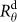, will give rise to a temperature gradient along the graphene ribbon27, while the heat resistance due to the e-p scattering,, dissipates heat uniformly. When the e-p pathway is dominant, the electron temperature Te will be determined by the strength of the e-p scattering and nearly uniform along the sample. We now show that this is indeed true in our devices. For a uniform temperature, Te at different biases can be obtained from the resistance R based on the RT curve. We have measured RIdc in magnetic fields of 0, 1, 5 T (Fig. 2(b)). Although the resistances are very different in three fields, after converting R to Te and Idc to Joule power P, all data collapse onto a single TeP curve, shown in Fig. 2(c). The scaling works surprisingly well for the whole range of the bias. The same scaling was also found in other devices (see Supplementary Fig. S3 online). It is a strong evidence for the proposed thermal model in Fig. 2(a) and Joule heating being the origin of the nonlinear resistance. More confidence in the model can be gained by analyzing the thermal resistance for the two pathways. The contact resistances are about 20 kΩ, which would yield a total contact thermal resistance of 273 K/nW for two contacts, according to the Wiedemann-Franz law. The total thermal resistance of the device is Rθ = dTe/dP ≈ 58 K/nW when TeT0, much less than the contact thermal resistance. As Te increases, Rθ sharply reduces. Therefore, the dissipation is mainly dominated by e-p scattering.

It becomes clear that the electron gas in our disordered graphene device is heated by the bias current. The electron temperature increases nearly uniformly along the device, leading to a decreasing resistance. We can calculate the electrical responsivity of the bolometer, which is the ratio of the voltage response to the Joule power29. In Fig. 3(a), the slope of the resistance R versus P, dR/dP, is plotted against P. The resistance sensitively responses to P, following a dR/dP P−2.3 behavior. When P < 0.01 nW, dR/dP is over 1 MΩ/nW. Using an a.c. excitation current of 2 nA, at which no appreciable heating occurs, we obtain a responsivity of 2 × 106 V/W at 1.5 K. The responsivity can be greatly improved if the temperature is to be further decreased, as the VRH resistance diverges at low temperature.

The responsivity of a graphene HEB depends mainly on two factors. One is the e-p coupling strength, which determines the amplitude of the temperature increase in response to a certain power input. The other is the voltage response to the temperature change, which is the sensitivity of graphene as a thermometer. In Fig. 1(e), it has been shown that disordered graphene exhibits a strong temperature coefficient. The temperature coefficient stems from localization of electrons by disorder and follows the VRH behavior. By varying the degree of disorder, the coefficient can be significantly tuned. We have measured devices of graphene films with sheet resistances ρ ranged from 30 to 350 kΩ. The change of the resistance in response to the power, dρ/dP, at P = 10 pW is plotted as a function of ρ in Fig. 3(b). It linearly follows ρ. The highest value in our experiment reaches 6 MΩ/nW. The corresponding responsivity of the device, which is 2.5 μm long and 5 μm wide, is 6 × 106 V/W at an excitation current of 2 nA.

To test the actual bolometric response of the device, we employed a red LED to illuminate the device. The LED emits light with a peak light wavelength of 650 nm. Since the whole device is under uniform and constant illumination, photovoltaic and photothermoelectric signals will not be picked up by the lock-in (See Method). Considering a multilayer structure, i.e., graphene/BN/SiO2/Si, the transfer matrix of the structure is deduced30 and the absorbed power by graphene is estimated to be 40 pW (2.7% absorptance, see the Supplementary online). With an excitation current of 1 nA, the voltage responses ΔV at different temperatures are plotted in Fig. 3(c). As a comparison, the responses to the same amount of Joule power are also plotted. We find a good qualitative agreement between two responses, which justifies our characterization of the bolometer using Joule heating13.

## Discussion

We have shown that the thermal dissipation pathway is mainly dominated by e-p scattering. Therefore, the thermal resistance due to e-p scattering can be computed by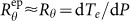. Fig. 4(a) shows the bias dependence of resistance at different temperatures. We can convert the RVdc plot into a TeP plot and then numerically compute the derivative dTe/dP, as plotted in Fig. 4(b). logdisplays a linear dependence on log Te, which is expected by theoretical work on e-p scattering in graphene. By fitting the linearity, we have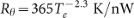. It has been predicted that the thermal resistance due to e-p scattering follows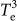when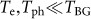and becomeswhen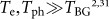2,31. Here, Tph is the phonon(lattice) temperature, while TBG is Bloch-Grüneisen temperature, defined as kBTBG = 2kFvph, where kF is the Fermi wave vector, vph the speed of sound5. For our sample with a measured Fermi level of 0.23 eV and the speed of sound vph = 2 × 104 m/s, TBG ≈ 102 K, much higher than Te and Tph. Considering a deformation potential D = 18 eV32 and Fermi velocity vF = 106 m/s, we find that for a graphene film with an area of 12.5 μm2,31. Note that these theories assume unscreened deformation potential and clean limit. Our result is in fact in good agreement with the theoretical prediction. We now turn to the role of disorders on e-p coupling. It has been proposed that disorder-assisted cooling pathway (supercollison) can be substantial for disordered graphene as it contributes to the energy loss rate besides conventional electron-phonon scattering33. Our experiment gives a larger thermal resistance comparing to the theoretical value, suggesting absence of supercollision. The result shown here is consistent with previous experimental studies13,19. Recently experiments have suggested suppercollision in graphene when34,35, while the normal e-p scattering dominates when, which does not contradict our results. The effect of disorder on e-p coupling can be considered an intriguing topic and it would be interesting to study. However, it is out of the scope in this work.

Having known the value of the thermal resistance, the response time τ of the bolometer can now be estimated by ρ ≈ RθCe, where Ce is the electronic heat capacity.when. At 1.5 K, the electronic heat capacity of our sample is about 2.4 × 10−20 J/K, resulting in τ ≈ 1.4 nS. Apparently, the intrinsic speed of graphene bolometer is extremely fast. However, in practical application, the actual response time is also limited by the RC time constant of the whole circuit, which is τ = RC. Here, R and C are the circuit resistance and capacitance, respectively. Take the sample above as an example. Considering the total area of four metal electrode pads and the graphene sample, the capacitance to the back gate is estimated to be 7 pF. The total capacitance of our measurement cables is roughly about 250 pF, while the total resistance, including the sample resistance and two contact resistances, is 94 kΩ. We then have τ ≈ 24 μs. Therefore, it is important to minimize the parasitic capacitance of the bolometer circuit and the resistance of graphene in order to approach the intrinsic speed of graphene HEBs.

Besides the responsivity, noise equivalent power (NEP) is a key factor that determines the performance of a bolometer. There are three noise sources, the measurement circuit noise, the thermal fluctuations and the Johnson noise14. The first one is extrinsic and depends on how the voltage measurement circuit is implemented. We now derive the latter two which are intrinsic to the device13. We have already obtained the thermal resistance Rθ ≈ 58 K/nW at 1.5 K. Thus, NEP by the thermal fluctuations is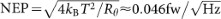. The Johnson noise is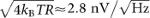for R = 54 kΩ plus the total contact resistance of 40 kΩ. The corresponding NEP is the noise divided by the responsivity,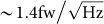. The NEP value on the device with the highest responsivity is indicated as.

When estimating the responsivity and NEP of our devices, we have used the absorbed power. Although this gives the ultimate performance of the device, it is useful to discuss the optical responsivity and NEP, estimated by taking the incident radiant power as the input. Taking into account the 2.7% absorptance of the device, we get an optical responsivity, 6 × 106 × 0.027 ≈ 1.6 × 105 V/W and a NEP of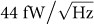. Therefore, it is important to increase the absorptance in order to obtain a better performance. This can be accomplished by using multilayer graphene, surface plasmonic enhancement36 or a microcavity37, although it is important to remember that the latter two introduce wavelength selectivity.

We now discuss the role of the BN layer. As we have explained, it increases the contact resistance, therefore reduces thermal dissipation by diffusion. As a result, the temperature is uniform along the device, which allows for straightforward derivation of the electron temperature and the thermal conductance and analysis of e-p scattering. However, the temperature uniformity is not a requirement for our bolometer, although it is helpful to gain the responsivity by suppression of heat dissipation into contacts. In addition to increasing the contact resistance, it can be achieved by enhancing the graphene resistivity or by modifying the device geometry, e.g., the ratio of the device area to the contact area. In fact, for disordered graphene, the resistivity is relatively high so that the heat dissipation through diffusion can be substantially reduced. As seen in the Supplementary Fig. S5 online, the devices made of disordered graphene film without the BN layer has shown a strong nonlinear resistance similar to the BN/graphene devices.

The basic structure of a disordered graphene HEB is comprised of only a graphene ribbon and two electrodes. It can be easily expanded to a detector array using conventional photo-lithography technique. In Fig. 5, we demonstrate the fabrication of an 2D array of HEB structures on a SiO2/Si substrate. Similar 2D array is the basic structure for 2D bolometric imaging, where each element works independently to provide a pixel. The graphene ribbon is 5 μm long and 2 μm wide. The fabrication procedure is similar to the one for making an individual device (see the Method). Although the e-beam lithography was used for this demonstration, photo-lithography is well suited for fabrication of the array. Note that the structure of the detector array has not been optimized, i.e., the optical fill-factor is very low. We want to point out that the simplicity of the device structure and the aspect ratio dependent responsivity and resistance, offer significant flexibility in device design to meet applications.

Compared with previous approaches, e.g., SdH oscillations, superconducting tunnel junctions and dual-gated bilayer graphene, disordered graphene provides a rather simple, yet effective scheme to implement a graphene HEB. The bolometer has an electrical responsivity of 6 × 106 V/W, over an order of magnitude higher than other approaches13,14. When considering the light absorptance of graphene devices, the optical responsivity is estimated to be 1.6 × 105 V/W. This value is much higher than 1 × 103 V/W for the dual-gated bilayer graphene device13, as our device does not require a metal top gate, which substantially reduces the absorptance. The derived value of NEP is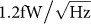(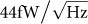optical NEP), also an order of magnitude lower than the previous result13. It does not require high mobility graphene films and dedicated micro-fabrication. The device characteristics, such as resistance, responsivity, can be tuned by adjusting the degree of disorder. It works at liquid Helium temperature and can potentially work at a higher temperature if disorder is enhanced. It is worth to note that multilayer can increase the absorptance and decrease the device resistance. Such a multilayer structure can be constructed by simple stacking in our case, while it is difficult, if not impossible, to achieve in other approaches.
The responsivity of disordered graphene HEB rivals Si bolometers and composite bolometers29. The NEP is much lower than that of Si bolometer,, but it is still orders of magnitude higher than the state-of-art superconducting edge transition bolometer operating at 40 mK38. However, we expect these parameters to have great improvement when the temperature is lowered. The most important advantage of graphene HEB is that they are unprecedentedly fast13,14, a few nanoseconds compared with a few milliseconds for others.